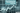# Leetcode Solution - Best Time to Buy and Sell Stock

July 06, 2021## Problem Statement

You are given an array prices where prices[i] is the price of a given stock on the ith day.

You want to maximize your profit by choosing a single day to buy one stock and choosing a different day in the future to sell that stock.

Return the maximum profit you can achieve from this transaction. If you cannot achieve any profit, return 0.

``````Example 1:

Input: prices = [7,1,5,3,6,4]
Output: 5
Explanation: Buy on day 2 (price = 1) and sell on day 5 (price = 6), profit = 6-1 = 5.
Note that buying on day 2 and selling on day 1 is not allowed because you must buy before you sell.

Example 2:

Input: prices = [7,6,4,3,1]
Output: 0
Explanation: In this case, no transactions are done and the max profit = 0.``````

## Brute Force - O(n^2)

``````public int maxProfit(int[] prices) {
int l=prices.length;
int maxProfit = 0;

for(int i=0; i<l-1; i++) {
for(int j=i+1; j<l; j++) {
int diff = prices[j] - prices[i];
if (diff > maxProfit) {
maxProfit = diff;
}
}
}

return maxProfit;
}``````

## Better Solution - O(n)

WWe can keep a track of minimum price as we iterate. And, calculate the profit till now. And we can also keep track of maximum profit so far.

The code is pretty simple.

``````public int maxProfit(int[] prices) {
int l=prices.length;

int maxProfit = 0;
int minPrice = prices;
for(int i=1; i<l; i++) {
maxProfit = Math.max(maxProfit, prices[i]-minPrice);
minPrice = Math.min(minPrice, prices[i]);
}

return maxProfit;
}``````